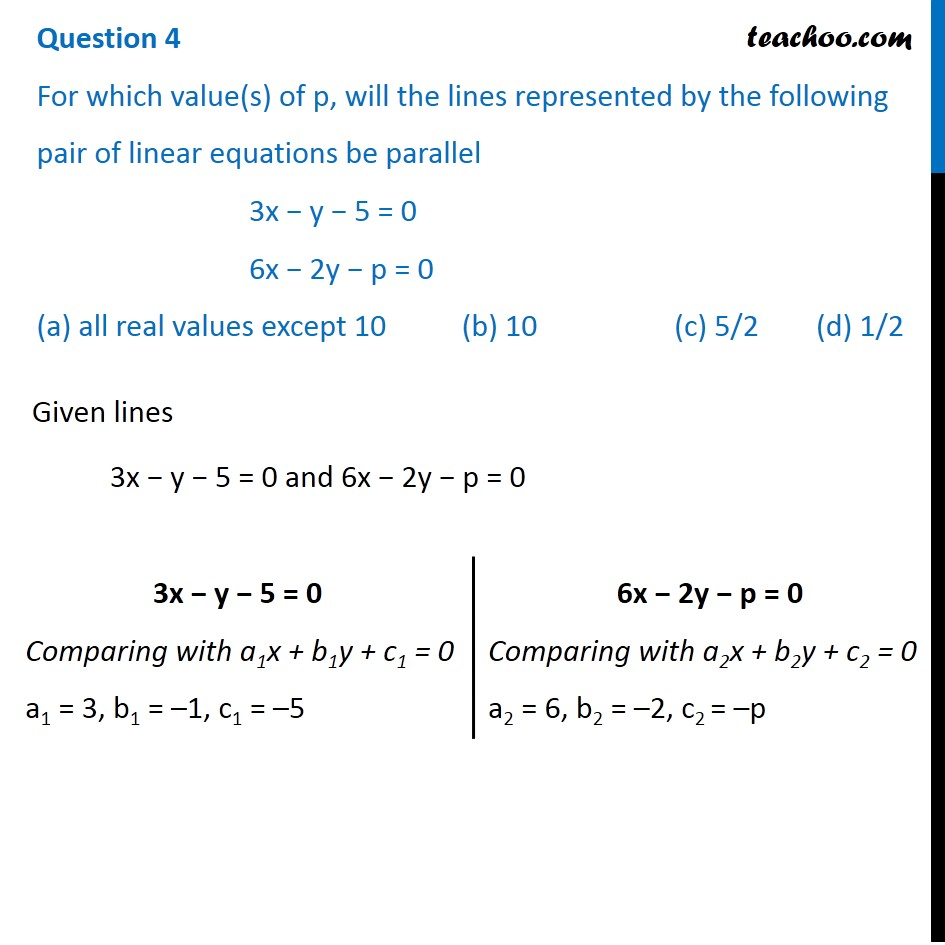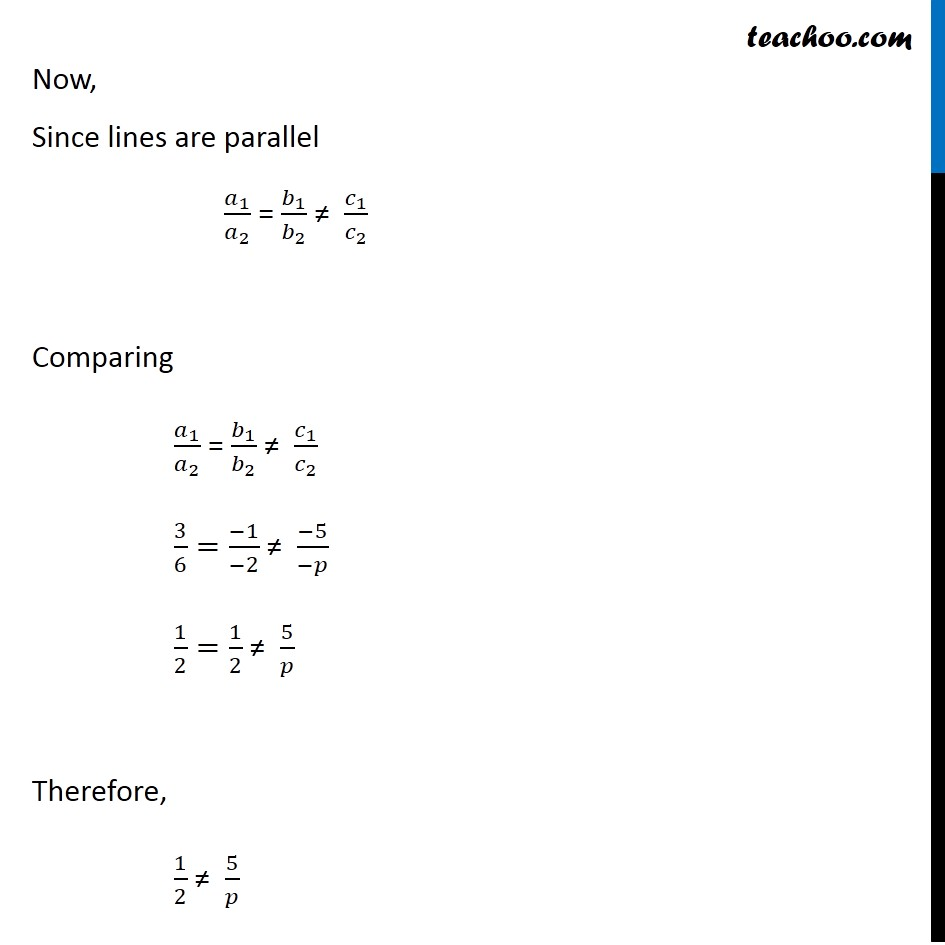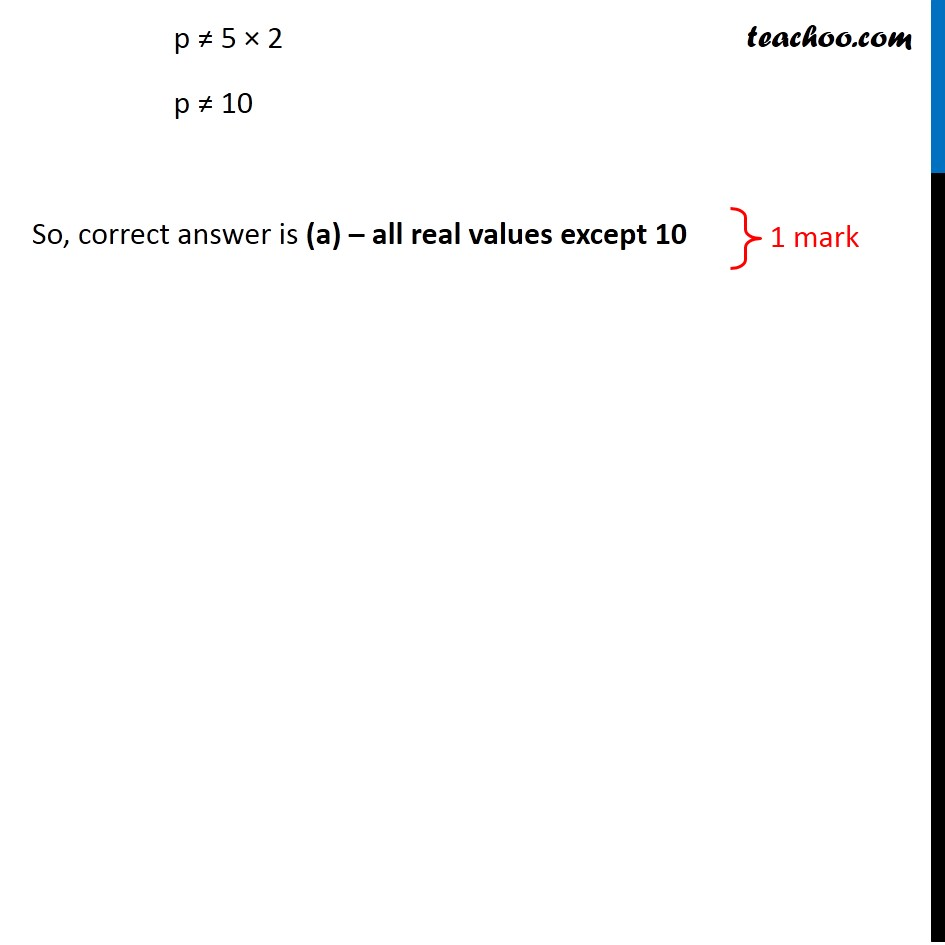## (a) all real values except 10  (b) 10  (c) 5/2  (d) 1/2Subscribe to our Youtube Channel - https://you.tube/teachoo

1. Class 10
2. Solutions of Sample Papers for Class 10 Boards
3. CBSE Class 10 Sample Paper for 2020 Boards - Maths Standard

Transcript

Question 4 For which value(s) of p, will the lines represented by the following pair of linear equations be parallel 3x − y − 5 = 0 6x − 2y − p = 0 (a) all real values except 10 (b) 10 (c) 5/2 (d) 1/2 Given lines 3x − y − 5 = 0 and 6x − 2y − p = 0 3x − y − 5 = 0 Comparing with a1x + b1y + c1 = 0 a1 = 3, b1 = –1, c1 = –5 6x − 2y − p = 0 Comparing with a2x + b2y + c2 = 0 a2 = 6, b2 = –2, c2 = –p Now, Since lines are parallel 𝑎_1/𝑎_2 = 𝑏_1/𝑏_2 ≠ 𝑐_1/𝑐_2 Comparing 𝑎_1/𝑎_2 = 𝑏_1/𝑏_2 ≠ 𝑐_1/𝑐_2 3/6=(−1)/(−2) ≠ (−5)/(−𝑝) 1/2=1/2 ≠ 5/𝑝 Therefore, 1/2 ≠ 5/𝑝 p ≠ 5 × 2 p ≠ 10 So, correct answer is (a) – all real values except 10

CBSE Class 10 Sample Paper for 2020 Boards - Maths Standard

Class 10
Solutions of Sample Papers for Class 10 Boards